# Factor Quadratic by Grid Method Explained

When factoring a quadratic expressionusing the grid method, we assume thatanddo not share any common factors (other than 1), and we assume that our expression does indeed factor, that is, there exist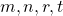such thatGiven the valuesand, how does the grid method correctly calculateand?

Since HCF, neither binomial will have a common factor: HCFand HCF.

By expanding the right hand side, we haveIt is immediately apparent thatand, however this does not help us calculate the values.

We also notice when we multiply two binomials of this kind that there are twoterms.

Let us break the our given value ofinto two values,and, where: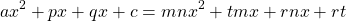# Calculating p, q given a, b, c

Let’s put this information into the grid format.

Given thatwe have: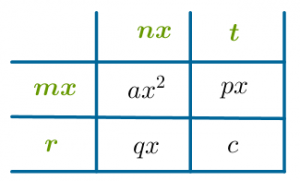Given thatwe have: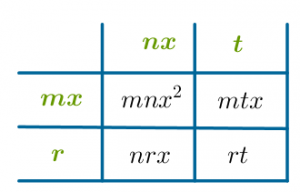In the second version, notice that when we multiply the two diagonals, we have the same expression:Therefore, from the first grid we haveWhich leads us to conclude.

We already know that(that is how we definedand).

This leads us to derive values foranddirectly from the coefficientsand: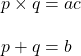If there are no integer solutions forand, then the values m, n, r, t are not integers. Our quadratic expression does not factor with integers.

By solving this system to calculateandwe can fill in the two by two grid:# Calculating m, n, r, t

At this point in the process, valuesandare unknown, however the values in the grid are complete. In terms of, the two by two grid is:Let’s consider the first row (any row or column will do)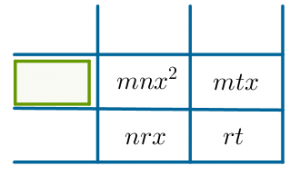Notice thatis common to both terms in this row. Also, as we noted in the beginning, HCF, thereforeis actually the highest common factor in this row.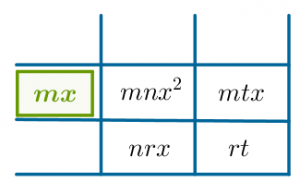Now let’s consider the first column. By a similar argument, we notice thatis the HCF of this column (because HCF). We also notice that we needto multiply withto get the required product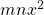:We continue in this way, drawing out the required factor in each row and column: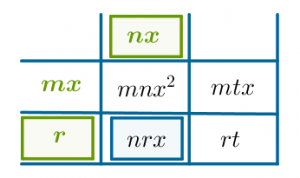Beginning with the valuesand, we have calculatedand.

The example once again:This grid above shows the valuesand. By drawing out the HCF of each row/column, we getand: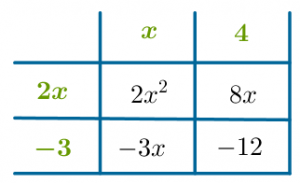Here,;,and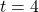.